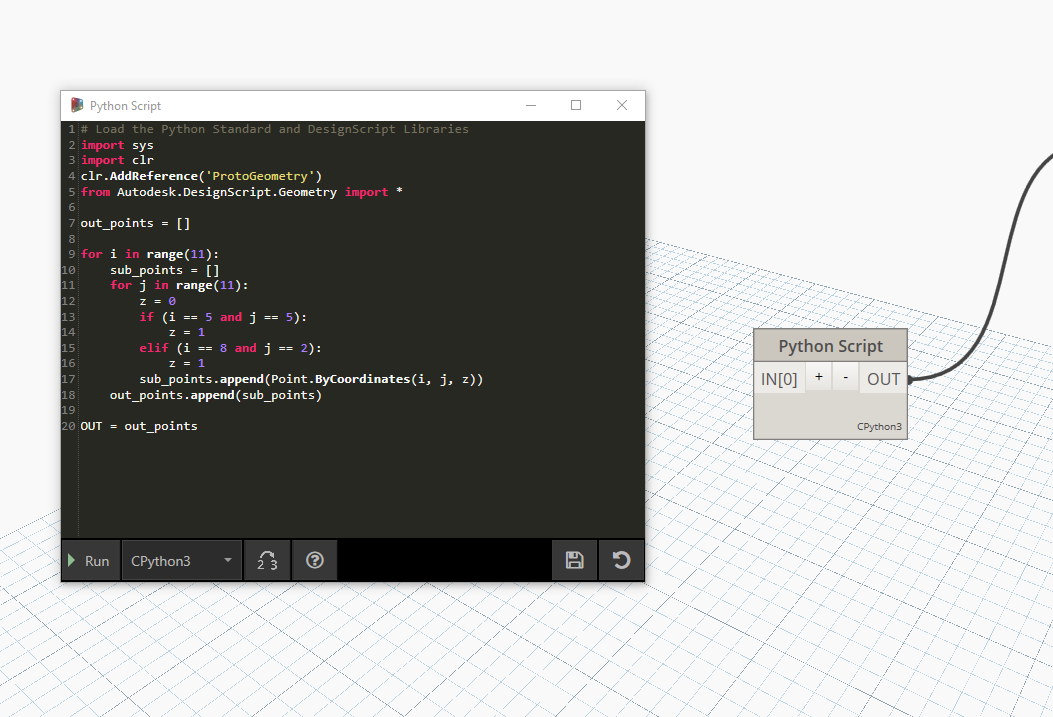# Python Point Generators

The following Python scripts generate point arrays for several examples. They should be pasted into a Python Script node as follows:python_points_1

``````out_points = []

for i in range(11):
sub_points = []
for j in range(11):
z = 0
if (i == 5 and j == 5):
z = 1
elif (i == 8 and j == 2):
z = 1

OUT = out_points
``````

python_points_2

``````out_points = []

for i in range(11):
z = 0
if (i == 2):
z = 1

OUT = out_points
``````

python_points_3

``````out_points = []

for i in range(11):
z = 0
if (i == 7):
z = -1

OUT = out_points
``````

python_points_4

``````out_points = []

for i in range(11):
z = 0
if (i == 5):
z = 1

OUT = out_points
``````

python_points_5

``````out_points = []

for i in range(11):
sub_points = []
for j in range(11):
z = 0
if (i == 1 and j == 1):
z = 2
elif (i == 8 and j == 1):
z = 2
elif (i == 2 and j == 6):
z = 2